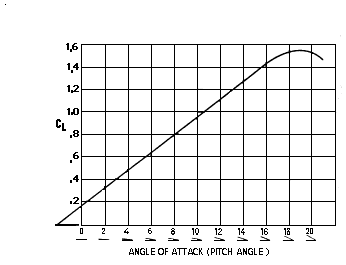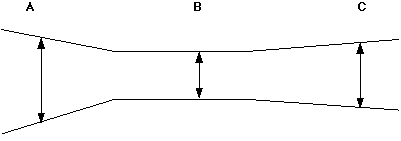## Pengalaman Belajar Fisika di SMAN BI 1 Banjar Ada Apa Dengan Fisika? Lift Formula

SUBJECT: Aeronautics
TOPIC: Lift
DESCRIPTION: A set of problems dealing with the aerodynamic lift equation.
CONTRIBUTED BY: Carol Hodanbosi
EDITED BY: Jonathan G. Fairman - August 1996

#### Purpose:

To understand for lift formula that determines aircraft lift capabilities.

#### Concept:

An aircraft's lift capabilities can be measured from the following formula:
L = (1/2) d v2 s CL
• L = Lift, which must equal the airplane's weight in pounds
• d = density of the air. This will change due to altitude. These values can be found in a I.C.A.O. Standard Atmosphere Table.
• v = velocity of an aircraft expressed in feet per second
• s = the wing area of an aircraft in square feet
• CL = Coefficient of lift , which is determined by the type of airfoil and angle of attack.
The angle of attack and CL are related and can be found using a Velocity Relationship Curve Graph (see Chart B below).

#### Exercises

1. You are flying an F-117A fully equipped, which means that your aircraft weighs 52,500 pounds. You want to maintain equilibrium in straight and level flight at an altitude of 30,000 feet, cruising at 400 knots to conserve fuel. The aircraft's wing area is 1,140 square feet. At what angle of attack should the F-117A be set to maintain equilibrium? ** Additional information you need to know to solve this problem:
How are knots related to mph to ft/s? See conversion below
What is air density at 30,000 feet? See following chart (I.C.A.O.). chart A
What is the relationship between CL and angle of attack?
Use following Chart B to determine this value.1 knot = 1 nautical mile per hour = 6,076 ft per hour
1 mph = 1 mile per hour = 5,280 feet per hour
2. To maintain an altitude of 45,000 feet at a constant speed requires an angle of attack of 4° . Your F-22 weighs 56,450 pounds, with a wing area of 830 square feet. To stay in level flight , what should your air speed indicate? The new F-22 air speed is indicated in Mach speed, not in knots. ** Additional information you need to know to solve this problem:
How does Mach speed convert from feet per second or from knots?
An angle of attack of 4° converts to what CL?
What is the density of air at 45,000 feet?
Mach 1 is the speed of sound, which varies with the air density. See the following I.C.A.O. chart (chart A) below. Mach 2 is twice the speed of sound, Mach 3 is three times the speed of sound.
Use the Velocity Relationship Curve Graph chart below, Chart B, to find the appropriate CL from the given angle of attack.
3. At what velocity should you be traveling in the F-22 to maintain an 0° angle of attack at an altitude of 42,000 feet? Your weight is 57,600 pounds
4. Using the same plane (F-22) the same weight (57,600 pounds) and angle of attack, what should be its cruising speed at 36,000 feet? While descending from 42,000 feet to 36,000 feet the plane covers a horizontal distance of 40 nautical miles. What is the decent angle in degrees?

Chart A
I.C.A.O. Standard Atmosphere Table
```
Altitude          Density     Speed of Sound
(Feet)             (d)         (Knots)
0          .002377          661.7
1,000          .002308          659.5
2,000          .002241          657.2
3,000          .002175          654.9
4,000          .002111          652.6
5,000          .002048          650.3
6,000          .001987          647.9
7,000          .001927          645.6
8,000          .001868          643.3
9,000          .001811          640.9
10,000          .001755          638.6
15,000          .001496          626.7
20,000          .001266          614.6
25,000          .001065          602.2
30,000          .000889          589.5
35,000          .000737          576.6
36,089*         .000706          573.8
40,000          .000585          573.8
45,000          .000460          573.8
50,000          .000362          573.8
55,000          .000285          573.8```
* Geopotential of Tropopause

Chart B
Velocity Relationship Curve Graph## Pengalaman Belajar Fisika di SMAN BI 1 Banjar Ada Apa Dengan Fisika?Experiment on Fluids: Finding the Velocity of a Fluid in a Confined Container

SUBJECT: Aeronautics
TOPIC: Fluid Velocity
DESCRIPTION: A set of mathematics problems dealing with fluid velocity.
CONTRIBUTED BY: Carol Hodanbosi
EDITED BY: Jonathan G. Fairman - August 1996

#### Purpose:

To calculate the velocity of a confined fluid, given the cross-section area and velocity of another region.

#### Concept:

The drawing below is a cross-section of a circular cone attached to a circular cylinder.When a fluid (liquid or gas) is in a confined space, with no change in pressure or temperature, one can use the equation of continuity to find the velocity of the fluid if one knows the cross-section area and velocity in one of the regions. The formula for this is A1*V1 = A2*V2, where A is the cross-section area of one location and V is the velocity for that location.

#### Analysis:

Given three different locations in a confined container, A, B, and C, all having different radii, can you find the other two velocities of the fluid, if the velocity at A is given?

1. If the cross-section at A has a radius of 6 meters, can you find the area of the slice through the cone
(Area = pi * r 2)?
2. If the velocity of the fluid at location A is 10.0 m/s, and the radius at location B is 4.2 meters, can you find the velocity at location B?
3. If the velocity at location C is 8.6 m/s, can you find the radius at location C?
4. If the radius of a fourth location, D, is one-half the radius of A , how would the velocity at location D compare to the velocity of the fluid at A? If D had one-third the radius of A, compare the velocity of the fluid at D to A. Explain your reasoning showing calculations.

Sumber:

NASA

## Tuesday, 18 July 2006

### Fisika SMA

Pengalaman Belajar Fisika di SMAN BI 1 Banjar

## Fluids Pressure and Depth

SUBJECT: Aeronautics
TOPIC: Hydrostatic Pressure
DESCRIPTION: A set of mathematics problems dealing with hydrostatics.
CONTRIBUTED BY: Carol Hodanbosi
EDITED BY: Jonathan G. Fairman - August 1996

A fluid is a substance that flows easily. Gases and liquids are fluids, although sometimes the dividing line between liquids and solids is not always clear. Because of their ability to flow, fluids can exert buoyant forces, multiply forces in a hydraulic systems, allow aircraft to fly and ships to float.
The topic that this page will explore will be pressure and depth. If a fluid is within a container then the depth of an object placed in that fluid can be measured. The deeper the object is placed in the fluid, the more pressure it experiences. This is because is the weight of the fluid above it. The more dense the fluid above it, the more pressure is exerted on the object that is submerged, due to the weight of the fluid.
The formula that gives the P pressure on an object submerged in a fluid is:

P = r * g * h
where
• r (rho) is the density of the fluid,
• g is the acceleration of gravity
• h is the height of the fluid above the object
If the container is open to the atmosphere above, the added pressure must be included if one is to find the total pressure on an object. The total pressure is the same as absolute pressure on pressure gauges readings, while the gauge pressure is the same as the fluid pressure alone, not including atmospheric pressure.

Ptotal = Patmosphere + Pfluid

Ptotal = Patmosphere + ( r * g * h )

A Pascal is the unit of pressure in the metric system. It represents 1 newton/m2
Example:
Find the pressure on a scuba diver when she is 12 meters below the surface of the ocean. Assume standard atmospheric conditions.
Solution:
The density of sea water is 1.03 X 10 3 kg/m3 and the atmospheric pressure is 1.01 x 105 N/m2.

Pfluid = r g h = (1.03 x10 3 kg/m3) (9.8 m/s2) (12 m) = 1.21 x 105 Newtons/m2
Ptotal = Patmosphere + Pfluid = (1.01 x 105) + (1.21 x 105 ) Pa = 2.22 x 10 2 kPa (kilo Pascals)

Exercises :

• What is the pressure experienced at a point on the bottom of a swimming pool 9 meters in depth? The density of water is 1.00 x 103 kg/m3.

• The interior of a submarine located at a depth of 45 meters is maintained at normal atmospheric conditions. Find the total force exerted on a 20 cm by 20 cm square window. Use the density of sea water given above.

• How many atmospheres is a depth of 100 meters of ocean water?

• If the weight density of pure water is 62 pounds/ft3, find the weight of water in a swimming pool whose dimensions are 20 ft by 10 ft by 6 feet.